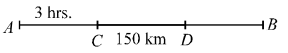Home » Aptitude » Speed, Time and Distance » Question

#### Speed, Time and Distance

1. A train met with an accident 3 hours after starting, which detains it for one hour, after which it proceeds at 75% of its original speed. It arrives at the destination 4 hours late. Had the accident taken place 150 km further along the railway line,
 the train would have arrived only 3 1 hours late. 2
Find the length of the trip and the original speed of the train.
1. 1100 km ; 100 kmph
2. 1200 km ; 100 kmph
3. 1200 km ; 90 kmph
4. 1600 km ; 90 kmph
##### Correct Option: B

Let A be the starting point, B the terminus. C and D are points where accidents take place.∴ 0.75 = 3 4

By travelling a 3/4 of its original speed, the train would take 4/3 of its usual time i.e., 1/3 more of the usual time.
∴  1/3 of the usual time taken to travel the distance CB.
= 4 – 1 = 3 hrs.     ...(i)
and 1/3 of the usual time taken to travel the distance
 DB = 3 1 − 1 = 2 1 hrs.   ...(ii) 2 2

Subtracting equation (ii) from (i)
we can write,
1/3 of the usual time taken to travel the distance
 CD = 3 − 2 1 = 1 hr. 2 2

∴  Usual time taken to travel
 CD (150 km) = 1 2 1 3

 = 3 hr. 2

Usual speed of the train
 = 3 = 9 hrs. 1 3

Total time = 3 + 9 = 12 hrs.
∴  Length of the trip = 12 × 100 = 1200 km.